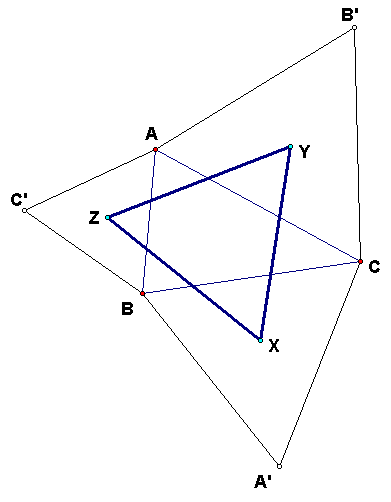## Napoleon's Theorem

This is a theorem attributed by legend to Napoleon Bonaparte.  It is rather doubtful that the Emperor actually discovered this theorem, but it is true that he was interested in mathematics.  He established such institutions as the Ecole Polytechnique with a view to training military engineers, but these institutions benefited mathematics greatly. French mathematicians made many important discoveries at the turn of the Eighteenth to the Nineteenth Century.

### Statement of Napoleon's Theorem

For any triangle ABC, build equilateral triangles on the sides.  (More precisely, for a side such as AB, construct an equilateral triangle ABC', with C and C' on opposite sides of line AB; do the same for the other two sides.). Then if the centers of the equilateral triangles are X, Y, Z, the triangle XYZ is equilateral.